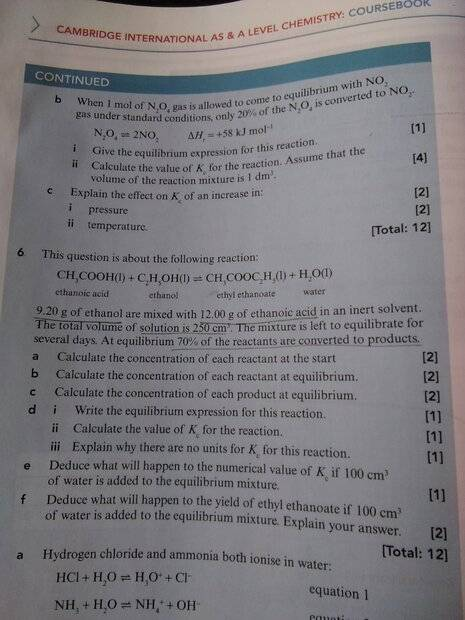# Finding equilibrium expression for an equilibrium equation

• Chemistry
• haha0p1
haha0p1
Homework Statement
Write the equilibrium expression for this reaction
Relevant Equations
Kc=(product A)×(product B)
----------------------------------------
(Reactant A)×(Reactant B)
In the d(i) part, we are asked to write an equilibrium constant. It was mentioned in the chapter that solids and liquids will not be added in the equation. So, I think that there is not going to be any Expression for this reaction as all the reactants and products are liquids. Is my answer right?Last edited:

Mentor
Solids and immiscible liquids.

Homework Helper
Gold Member
I suggest you use square brackets in your above expressions to remind yourself that you are dealing with molarities.

jeanpinto844
You need to convert the masses of your reactants into moles using their respective molar masses. You can then find the initial concentrations from the moles and the given volume. The reactions shows the reactants as liquids but they are being dissolved in a solvent which then makes them aqueous. You need the values for part (a) in order to complete the entire problem.

Mayhem
There's always an expression. The reason we don't include materials with certain properties is because their activities evaluate to 1, i.e. something multiplied by 1 is itself, so it's not written out. The confusion likely arises from the fact that we usually only include (g) and (aq) samples in these equations, since a lot of problems are concerned with dissolved gases, salts or acid/bases. However, this problem deals with a mixture of liquids rather than what we would usually consider a solution (i.e. something solid or gaseous dissolved).